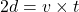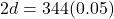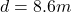Sound travels through air at 343 m/s at 20 °C. A bat emits an ultrasonic squeak and hears the echo 0.05 second later . How far away was the

Question

Sound travels through air at 343 m/s at 20 °C. A bat emits an ultrasonic squeak and hears the echo 0.05 second later . How far away was the object that reflected it?

in progress 0
5 months 2021-08-04T07:18:22+00:00 1 Answers 6 views 0

Distance of the object is 8.6 m

Explanation:

As we know that the speed of sound at t degree C is given as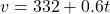here we know that the temperature is

t = 20 degree C

so we have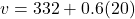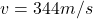now we know that bat heard the echo of sound in 0.05 s

so the to and fro distance of the object is d + d = 2d

so we have